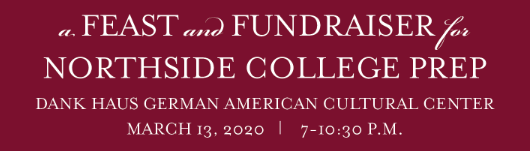# 2020 SPRING BENEFIT

## Friday, March 13 | 7:00 - 10:30 pm

 /* styles */ Join us for a festive evening of food, drink, and live entertainment to benefit Northside College Prep! Mingle with fellow Northside parents and faculty as we raise essential funding for our students - and celebrate cultural diversity with a buffet of local favorites and craft spirits. DANK Haus, 4740 N. Western Ave.

# Don't Delay! Early Bird Tickets on Sale Only Through Sunday, February 23

Early Bird Tickets - only \$100

## Parent Sponsorship Tickets are Also Available:

20th Anniversary Circle (includes four tickets with reserved seating plus two teacher tickets) - \$1200
Benefactor (includes two tickets plus two teacher tickets) - \$900
Supporter (includes two tickets plus one teacher ticket) - \$600
Friend (includes two tickets) - \$300

All Parent Sponsors will be recognized at the Benefit, online at the FON website, and in This Week at Northside, the FON weekly eblast.

# Visit northside.givesmart.com to purchase tickets

## Sponsor NCP Teachers and Staff to attend:

▪ Buy one NCP staff ticket for \$65
▪ Buy five NCP staff tickets for \$300
▪ Sponsor ten NCP staff tickets for \$550

## Event Details and Tickets

Guests at this adults-only event will enjoy:

▪ Open Beer & Wine Bar
▪ Complimentary hand-crafted Mustang cocktails
▪ Dinner & Dessert Buffet including food from some of Chicago's best restaurants
▪ Silent & Live Auctions
▪ Paddle Raise to fund a new Turf Field
▪ Live Music by Northside Parent Matt Farmer and his band
▪ ... and more!
 ▪ Open Beer & Wine Bar
 ▪ Complimentary hand-crafted Mustang cocktails
 ▪ Dinner & Dessert Buffet including food from some of Chicago's best restaurants
 ▪ Silent & Live Auctions
 ▪ Paddle Raise to fund a new Turf Field
 ▪ Live Music by Northside Parent Matt Farmer and his band
 ▪ ... and more!

# Northside Students say "Thank You" for the New Library

Every year, Friends of Northside partners with NCP Administration to identify an important project that needs financial support. In 2019, the Spring Benefit Paddle Raise raised over \$40,000 for library renovations. Since it's opening last year, the new library has been a central place for students to study, collaborate, relax, and socialize. Please watch this VIDEO created by Northside's own Mustang Reel video club.## Donations Requested!

Silent & Live Auction Items Needed: Donations to the silent and live auctions are needed. Please consider donating:

▪ Tickets to sporting events
▪ Professional services
▪ Vacation homes
▪ Hotel accommodations
▪ Special experiences
▪ Airline miles
 ▪ Tickets to sporting events
 ▪ Professional services
 ▪ Vacation homes
 ▪ Hotel accommodations
 ▪ Special experiences
 ▪ Airline miles

Click HERE for the donation form or email Kristen Werries Collier at kristenwerriescollier@gmail.com.

Parent Parties: NCP parents have the chance to pay for a spot at a variety of parties put on by other NCP parents with all proceeds going back to the school. Party hosts assume all costs of the parties they throw. Parent-run parties have included such themes as Moms & Margaritas, Backyard BBQs, Trivia Night, and more. Brainstorm your ideas and sign up to throw a party! Contact Christine Lee at chrissoolee@yahoo.com for more details and to sign up to host.

# Know a Great Restaurant?

## More Food Donations Needed for our Taste of Northside Buffet

We are looking for donations of a couple of more dinner items to join this fantastic lineup of restaurants. It could be from a favorite restaurant of yours or a restaurant where you may know the owner personally. We are simply asking for one dish, hot or cold, which could be served in taste portions to approximately 300 people. Friends of Northside will be happy to provide marketing for the restaurant before, during, and after the event. Please contact Matt Schechter at matthew.d.schechter@gmail.com if you have a recommendation. Here is the list of current donors for our event.

 ▪ King Pho
 /* styles */ All of these restaurants and businesses have generously donated their food and beverages to our event, which helps us to keep costs down so that the funds we raise go directly to programs and services for our students at Northside. Please support these great Chicago restaurants and tell them, "Thank you!"Corporate sponsorship contributions will be used to underwrite the cost of the 2020 Spring Benefit and enable Friends of Northside to provide more of its financial resources for programs and services for the school not covered by the CPS budget. For sponsorship opportunities, please contact Peter Beale-DelVecchio, peterbealedelvecchio@gmail.com. Click HERE for the sponsorship form.We are putting together a slideshow of our students participating in the many activities that make Northside special. If you have pictures you'd like to be considered for inclusion in the presentation, please forward them to Erika Schechter at erikaschechter@gmail.com. Or you can add them to this Google Folder.

 table div table+table+table+table+table+table+table+table+table+table+table+table+table+table+table+table+table+table+table+table div table{width:100%;padding:0}table div table+table+table+table+table+table+table+table+table+table+table+table+table+table+table+table+table+table+table+table div table img{width:96.23%;padding:0;float:none}table div table+table+table+table+table+table+table+table+table+table+table+table+table+table+table+table+table+table+table+table div table td{width:100%;padding:0 1.88% 18px}/* styles */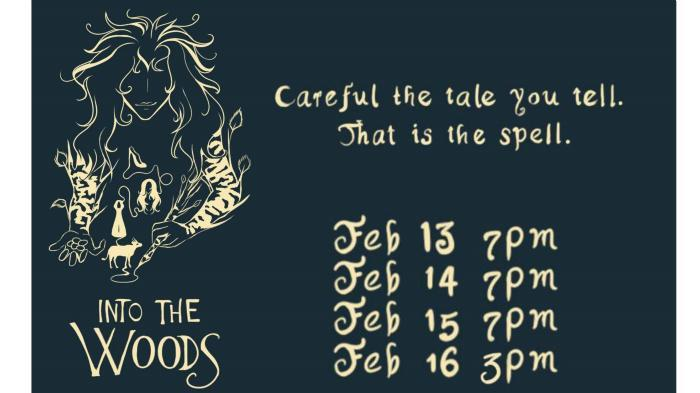# Spring Musical: Into the Woods

▪ Thursday, February 13 at 7:00 pm
▪ Friday, February 14 at 7:00 pm
▪ Saturday, February 15 at 7:00 pm
▪ Sunday, February 16 at 3:00 pm
 ▪ Thursday, February 13 at 7:00 pm
 ▪ Friday, February 14 at 7:00 pm
 ▪ Saturday, February 15 at 7:00 pm
 ▪ Sunday, February 16 at 3:00 pm
 table div table+table+table+table+table+table+table+table+table+table+table+table+table+table+table+table+table+table+table+table+table+table div table{width:100%;padding:0}table div table+table+table+table+table+table+table+table+table+table+table+table+table+table+table+table+table+table+table+table+table+table div table img{width:96.23%;padding:0;float:none}table div table+table+table+table+table+table+table+table+table+table+table+table+table+table+table+table+table+table+table+table+table+table div table td{width:100%;padding:0 1.88% 18px}/* styles */# This Week in Sports

Please come out and cheer on our mighty Mustangs at the following games:

▪ Monday, February 10, 5:45 pm (F) vs. Amundsen at NCP
▪ Tuesday, February 11, 5:30 pm (V) vs. Lake Forest Academy at NCP
▪ Thursday, February 13, 5:45 pm (F) vs. Lake View at NCP
▪ Friday, February 14, 5:00 pm (V) and 6:30 pm (SO) vs. Holy Trinity at NCP
▪ Sunday, February 16, 1:00 pm (V) and 2:30 pm (SO) vs. Yashiva at NCP
 ▪ Monday, February 10, 5:45 pm (F) vs. Amundsen at NCP
 ▪ Tuesday, February 11, 5:30 pm (V) vs. Lake Forest Academy at NCP
 ▪ Thursday, February 13, 5:45 pm (F) vs. Lake View at NCP
 ▪ Friday, February 14, 5:00 pm (V) and 6:30 pm (SO) vs. Holy Trinity at NCP
 ▪ Sunday, February 16, 1:00 pm (V) and 2:30 pm (SO) vs. Yashiva at NCP

▪ Monday, February 10, 4:30 pm (V) vs. Mather at NCP for Senior Day
▪ Tuesday, February 11, 5:00 pm (V) vs. North Grand at North Grand
▪ Thursday, February 13, 4:30 pm (V) vs. Von Steuben at NCP
 ▪ Monday, February 10, 4:30 pm (V) vs. Mather at NCP for Senior Day
 ▪ Tuesday, February 11, 5:00 pm (V) vs. North Grand at North Grand
 ▪ Thursday, February 13, 4:30 pm (V) vs. Von Steuben at NCP

Information subject to change.

Welcome to Mark David as our new freshman Men's basketball coach! Coach David comes to us from Hibbard school where he coaches soccer.

 table div table+table+table+table+table+table+table+table+table+table+table+table+table+table+table+table+table+table+table+table+table+table+table+table div table{width:100%;padding:0}table div table+table+table+table+table+table+table+table+table+table+table+table+table+table+table+table+table+table+table+table+table+table+table+table div table img{width:96.23%;padding:0;float:none}table div table+table+table+table+table+table+table+table+table+table+table+table+table+table+table+table+table+table+table+table+table+table+table+table div table td{width:100%;padding:0 1.88% 18px}/* styles */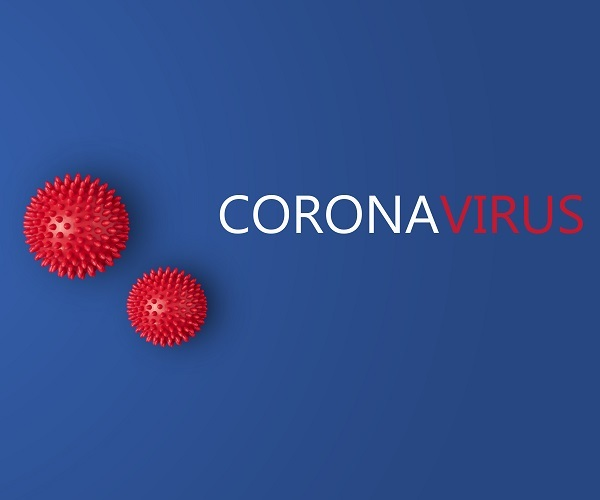# CPS Released Updated Recommendations Regarding the Coronavirus

Please click for the letter in English, Spanish, and Chinese.

For the most reliable information, please visit www.chicago.gov/2019-nCoV or www.cdc.gov/coronavirus/2019-ncov. If you have any questions or concerns, please contact the CDPH Coronavirus hotline at 312-746-4835 or email coronavirus@chicago.gov. You may also reach out to the CPS Office of Student Health & Wellness at oshw@cps.edu.

 table div table+table+table+table+table+table+table+table+table+table+table+table+table+table+table+table+table+table+table+table+table+table+table+table+table+table div table{width:100%;padding:0}table div table+table+table+table+table+table+table+table+table+table+table+table+table+table+table+table+table+table+table+table+table+table+table+table+table+table div table img{width:96.23%;padding:0;float:none}table div table+table+table+table+table+table+table+table+table+table+table+table+table+table+table+table+table+table+table+table+table+table+table+table+table+table div table td{width:100%;padding:0 1.88% 18px}/* styles */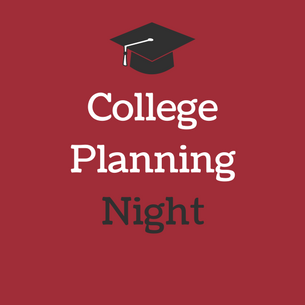# College Planning Night

Thank you, Junior Students and Parents, for attending the Northside Prep, Junior College Planning Night on Tuesday, February 4. The counseling team is looking forward to discussing your postsecondary plans during upcoming College Conferences. For more details about scheduling college conferences, contact your respective college counselor. To improve future Northside College Prep Counseling Department presentations, activities, and resources click HERE to complete the College Planning Survey.

To access the handouts that were provided during College Planning Night, click on the links below:

 table div table+table+table+table+table+table+table+table+table+table+table+table+table+table+table+table+table+table+table+table+table+table+table+table+table+table+table+table div table{width:100%;padding:0}table div table+table+table+table+table+table+table+table+table+table+table+table+table+table+table+table+table+table+table+table+table+table+table+table+table+table+table+table div table img{width:96.23%;padding:0;float:none}table div table+table+table+table+table+table+table+table+table+table+table+table+table+table+table+table+table+table+table+table+table+table+table+table+table+table+table+table div table td{width:100%;padding:0 1.88% 18px}/* styles */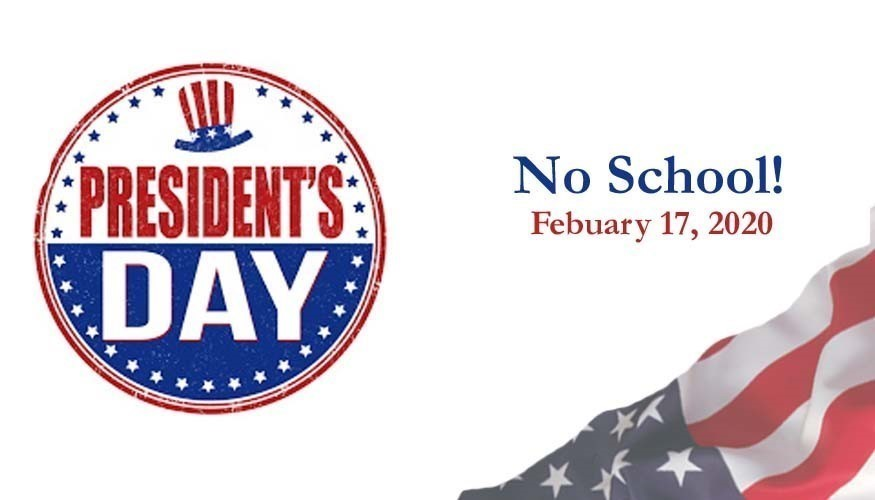# President's Day

Monday, February 17
No School

 table div table+table+table+table+table+table+table+table+table+table+table+table+table+table+table+table+table+table+table+table+table+table+table+table+table+table+table+table+table+table div table{width:100%;padding:0}table div table+table+table+table+table+table+table+table+table+table+table+table+table+table+table+table+table+table+table+table+table+table+table+table+table+table+table+table+table+table div table img{width:96.23%;padding:0;float:none}table div table+table+table+table+table+table+table+table+table+table+table+table+table+table+table+table+table+table+table+table+table+table+table+table+table+table+table+table+table+table div table td{width:100%;padding:0 1.88% 18px}/* styles */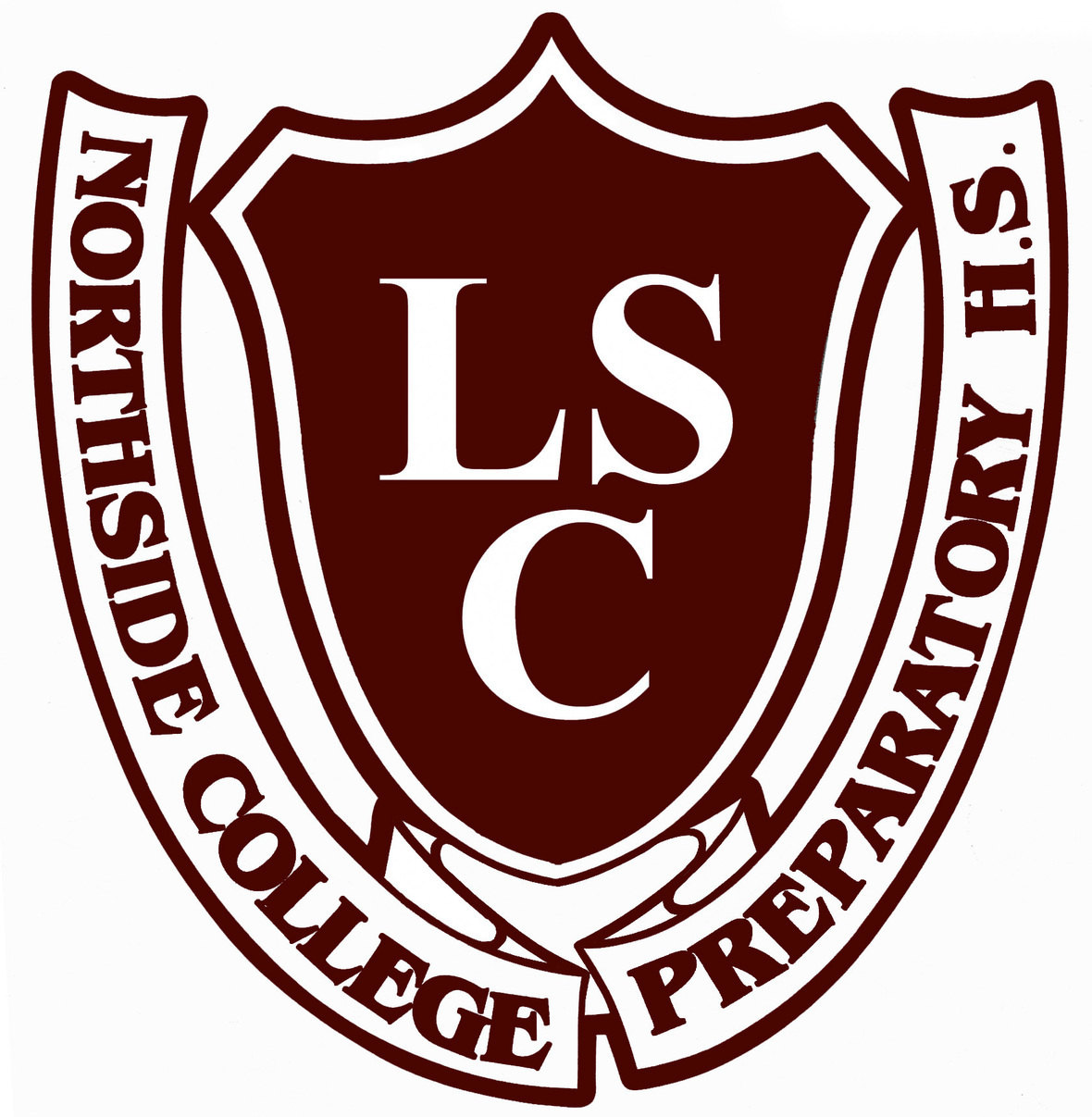# LSC Meeting

Tuesday, February 18
6:30 pm
Faculty Lounge

 table div table+table+table+table+table+table+table+table+table+table+table+table+table+table+table+table+table+table+table+table+table+table+table+table+table+table+table+table+table+table+table+table div table{width:100%;padding:0}table div table+table+table+table+table+table+table+table+table+table+table+table+table+table+table+table+table+table+table+table+table+table+table+table+table+table+table+table+table+table+table+table div table img{width:96.23%;padding:0;float:none}table div table+table+table+table+table+table+table+table+table+table+table+table+table+table+table+table+table+table+table+table+table+table+table+table+table+table+table+table+table+table+table+table div table td{width:100%;padding:0 1.88% 18px}/* styles */# Be a Voice for Your School - Run for an LSC Position

## Seeking Candidates for our 2020-2022 Local School Council (LSC)

Be a voice for Northside! The election for next year’s (2020-2022) Local School Council is in spring 2020. Candidates are needed for all six parent representatives and two community representative positions. Please consider running for one of these important posts and participate in the governance of your child’s school. Additional information is available in this cover letter from CPS in English and in Spanish. Forms are available in the school office and on the CPS website.

▪ Parent reps must have a student enrolled at Northside this year, as well as while they are in office.

▪ Community reps may not have a student enrolled during their term and must live on the North Side of the city.

Interested candidates can nominate themselves for a position by returning the completed form to the school office. Candidates may also provide a short statement of their qualifications and why they want to be on the LSC. Nomination forms must be turned in to Northside by March 5 at 3:00 pm. A Candidate Forum will be held prior to elections - with date TBD. The election is on Thursday, April 23, with voting conducted during report card pick-up.

 table div table+table+table+table+table+table+table+table+table+table+table+table+table+table+table+table+table+table+table+table+table+table+table+table+table+table+table+table+table+table+table+table+table+table div table{width:100%;padding:0}table div table+table+table+table+table+table+table+table+table+table+table+table+table+table+table+table+table+table+table+table+table+table+table+table+table+table+table+table+table+table+table+table+table+table div table img{width:96.23%;padding:0;float:none}table div table+table+table+table+table+table+table+table+table+table+table+table+table+table+table+table+table+table+table+table+table+table+table+table+table+table+table+table+table+table+table+table+table+table div table td{width:100%;padding:0 1.88% 18px}/* styles */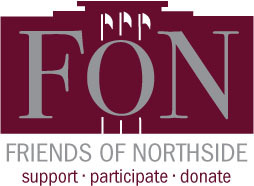# Friends of Northside Meeting

Tuesday, February 25
7:00 pm
Faculty Lounge

 table div table+table+table+table+table+table+table+table+table+table+table+table+table+table+table+table+table+table+table+table+table+table+table+table+table+table+table+table+table+table+table+table+table+table+table+table div table{width:100%;padding:0}table div table+table+table+table+table+table+table+table+table+table+table+table+table+table+table+table+table+table+table+table+table+table+table+table+table+table+table+table+table+table+table+table+table+table+table+table div table img{width:96.23%;padding:0;float:none}table div table+table+table+table+table+table+table+table+table+table+table+table+table+table+table+table+table+table+table+table+table+table+table+table+table+table+table+table+table+table+table+table+table+table+table+table div table td{width:100%;padding:0 1.88% 18px}/* styles */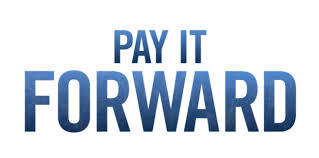# Volunteers Needed to Help the Pay It Forward Colloquium

Be a part of this amazing tradition! The Pay It Forward colloquium needs parent volutneers. If you're able to volunteer, please contact Mr. Blease, rblease@cps.edu or Dr. Eakin, epeakin@cps.edu.

Only ONE volunteer is needed for each event:

▪ Wednesday, February 26, 10:30 am-1:20 pm, event TBD
▪ Wednesday, March 4, 10:45am-1:00 pm, walk to Budlong Elementary School
▪ Wednesday, March 11, 8:00-11:30 am, bus chaperone to Feed My Starving Children in Schaumburg.
▪ Wednesday, March 25, 8:00-11:30 am, bus chaperone to Bernie's Books in Schaumburg
▪ Wednesday, April 1, 10:30 am-12:15 pm, walk to Covenant Senior Center
▪ Wednesday, May 20, 10:30 am-12:15 pm, walk to Covenant Senior Center
▪ Wednesday, June 3, 10:45 am-1:00 pm, walk to Budlong Elementary School
 ▪ Wednesday, February 26, 10:30 am-1:20 pm, event TBD
 ▪ Wednesday, March 4, 10:45am-1:00 pm, walk to Budlong Elementary School
 ▪ Wednesday, March 11, 8:00-11:30 am, bus chaperone to Feed My Starving Children in Schaumburg.
 ▪ Wednesday, March 25, 8:00-11:30 am, bus chaperone to Bernie's Books in Schaumburg
 ▪ Wednesday, April 1, 10:30 am-12:15 pm, walk to Covenant Senior Center
 ▪ Wednesday, May 20, 10:30 am-12:15 pm, walk to Covenant Senior Center
 ▪ Wednesday, June 3, 10:45 am-1:00 pm, walk to Budlong Elementary School
 table div table+table+table+table+table+table+table+table+table+table+table+table+table+table+table+table+table+table+table+table+table+table+table+table+table+table+table+table+table+table+table+table+table+table+table+table+table+table div table{width:100%;padding:0}table div table+table+table+table+table+table+table+table+table+table+table+table+table+table+table+table+table+table+table+table+table+table+table+table+table+table+table+table+table+table+table+table+table+table+table+table+table+table div table img{width:96.23%;padding:0;float:none}table div table+table+table+table+table+table+table+table+table+table+table+table+table+table+table+table+table+table+table+table+table+table+table+table+table+table+table+table+table+table+table+table+table+table+table+table+table+table div table td{width:100%;padding:0 1.88% 18px}/* styles */# Attention Parents & Friends of the Class of 2020!

To commemorate your student's accomplishments or declare your best wishes, The Stampede, Northside's yearbook, offers ad space in its upcoming edition.

The prices are as follows:
▪ Full Page - \$150
▪ Half Page - \$80
▪ Quarter Page - \$50

Please see this ORDER FORM for details. All materials are due by Friday, March 20.

Questions? Contact Marion McCreedy, Stampede adviser, at mkmccreedy@cps.edu.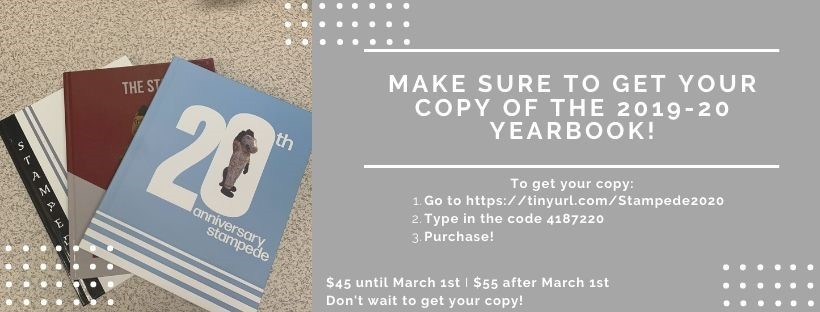## And While We're Talking About Yearbooks...

Order yours now to avoid the March 1 price increase!

To get your copy of the 2019-20 Stampede:
1. Go to: https://tinyurl.com/Stampede2020
2. Type in the code: 4187220
3. And purchase!

The price will be \$45 until March 1 and \$55 after March 1, so don't wait to get your copy!

 table div table+table+table+table+table+table+table+table+table+table+table+table+table+table+table+table+table+table+table+table+table+table+table+table+table+table+table+table+table+table+table+table+table+table+table+table+table+table+table+table+table+table+table div table{width:100%;padding:0}table div table+table+table+table+table+table+table+table+table+table+table+table+table+table+table+table+table+table+table+table+table+table+table+table+table+table+table+table+table+table+table+table+table+table+table+table+table+table+table+table+table+table+table div table img{width:96.23%;padding:0;float:none}table div table+table+table+table+table+table+table+table+table+table+table+table+table+table+table+table+table+table+table+table+table+table+table+table+table+table+table+table+table+table+table+table+table+table+table+table+table+table+table+table+table+table+table div table td{width:100%;padding:0 1.88% 18px}/* styles */# Parent Volunteers Needed to Help the Spanish Honor Society

## Annual Initiation Ceremony

Tuesday, March 10
6:45 pm - 8:30 pm
The Spanish Honor Society will hold its annual Initiation Ceremony and needs help from 2-3 parent volunteers to help serve cake and drinks in the cafeteria to the attendees.

## Dia de los Ninos

Saturday, April 25
8:00 am - 4:00 pm
The Spanish Honor Society is in need of 10, or more, parent volunteers for their Activity of the Year: Dia de los Ninos on Saturday, April 25 from 8:00 am-4:00 pm. HERE are the pictures from last year. There will be a bus that will pick us up at Northside at 8:00 am and will bring us back to Northside around 3:30 pm that day.

 table div table+table+table+table+table+table+table+table+table+table+table+table+table+table+table+table+table+table+table+table+table+table+table+table+table+table+table+table+table+table+table+table+table+table+table+table+table+table+table+table+table+table+table+table+table div table{width:100%;padding:0}table div table+table+table+table+table+table+table+table+table+table+table+table+table+table+table+table+table+table+table+table+table+table+table+table+table+table+table+table+table+table+table+table+table+table+table+table+table+table+table+table+table+table+table+table+table div table img{width:96.23%;padding:0;float:none}table div table+table+table+table+table+table+table+table+table+table+table+table+table+table+table+table+table+table+table+table+table+table+table+table+table+table+table+table+table+table+table+table+table+table+table+table+table+table+table+table+table+table+table+table+table div table td{width:100%;padding:0 1.88% 18px}/* styles */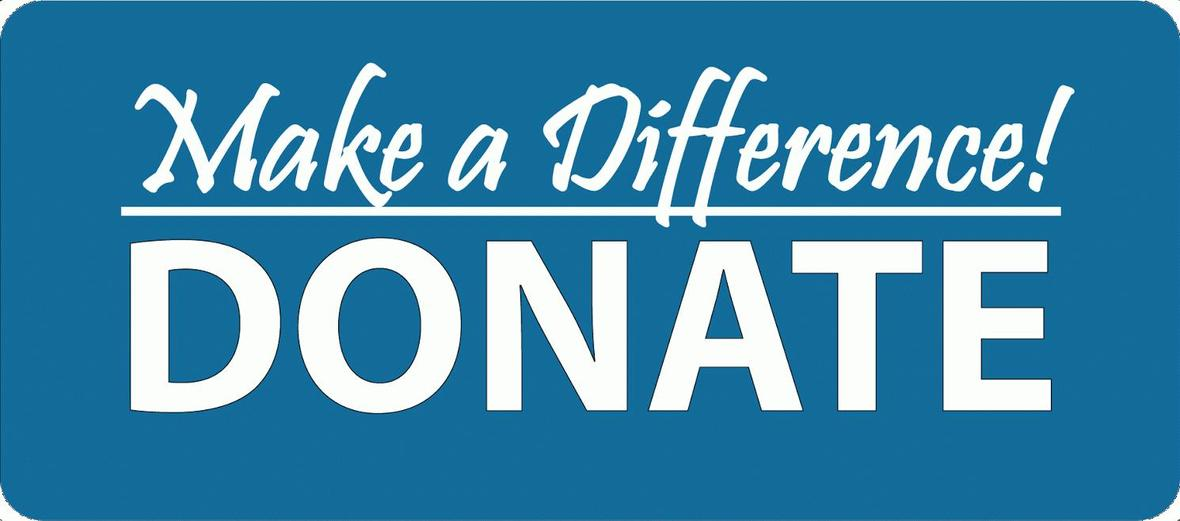# Donations Needed for Student Resource Closet (Kato's Corner)

Friends of Northside has a resource closet available to NCP students. We are in need of:

▪ Individual snacks such as granola bars, nuts, and dried fruit
▪ Body lotion
▪ Toothbrushes
▪ Shaving gel for women
▪ Athletic apparel for men and women (shirts, shorts, pants)
▪ Mittens and gloves
▪ Shampoo and conditioner
 ▪ Individual snacks such as granola bars, nuts, and dried fruit
 ▪ Body lotion
 ▪ Toothbrushes
 ▪ Shaving gel for women
 ▪ Athletic apparel for men and women (shirts, shorts, pants)
 ▪ Mittens and gloves
 ▪ Shampoo and conditioner

All items must be new and unopened. No used clothing will be accepted. Please bring all items to the Counseling Office and set outside of Ms. Matthews' office.

Contact Nancy Liljedahl with any questions.

 table div table+table+table+table+table+table+table+table+table+table+table+table+table+table+table+table+table+table+table+table+table+table+table+table+table+table+table+table+table+table+table+table+table+table+table+table+table+table+table+table+table+table+table+table+table+table+table+table div table{width:100%;padding:0}table div table+table+table+table+table+table+table+table+table+table+table+table+table+table+table+table+table+table+table+table+table+table+table+table+table+table+table+table+table+table+table+table+table+table+table+table+table+table+table+table+table+table+table+table+table+table+table+table div table img{width:96.23%;padding:0;float:none}table div table+table+table+table+table+table+table+table+table+table+table+table+table+table+table+table+table+table+table+table+table+table+table+table+table+table+table+table+table+table+table+table+table+table+table+table+table+table+table+table+table+table+table+table+table+table+table+table div table td{width:100%;padding:0 1.88% 18px}/* styles */Congratulations to Junior Guadalupe Quiñones and Senior Edgar Castillo for winning two amazing awards as members of the Spanish Honor Society.

▪ Edgar Castillo won the Joseph Adam Award of \$2,000.00.
▪ Guadalupe Quiñones won the Bertie Green Junior Travel Award, which includes an “all-inclusive trip" to Ecuador from July 20 – July 30.
 ▪ Edgar Castillo won the Joseph Adam Award of \$2,000.00.
 ▪ Guadalupe Quiñones won the Bertie Green Junior Travel Award, which includes an “all-inclusive trip" to Ecuador from July 20 – July 30.

The Spanish Honor Society is an academic honor society focused on Spanish language excellence in secondary education and promotes a continuity of interest in Spanish studies.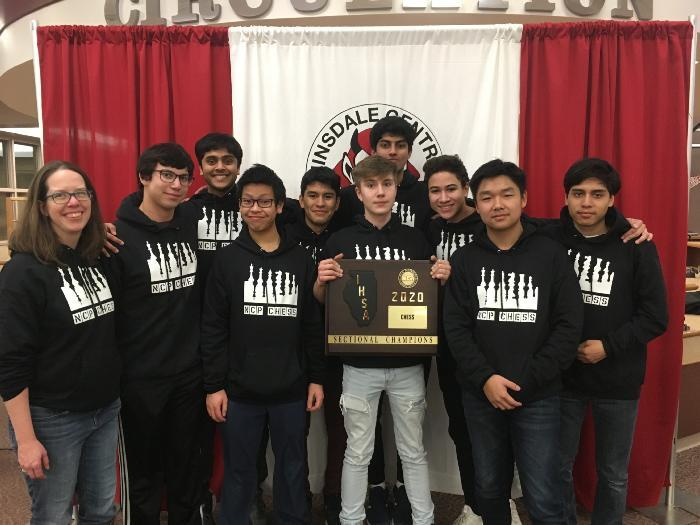# NCP Varsity Chess Team wins 1st Place at State Sectionals

Congratulations to the varsity chess team which won first place at the State Sectionals. The team went undefeated the whole day, winning all four rounds.

The varsity chess team is comprised of the following members:

Richard Lam, Aaron Mui, Umair Ahmed, Konrad Freymiller, Arya Desai, Zachary McCoy, Jake Jasmer, Oscar Rico, Alan Rico

# Do you have news or team results to share with the Northside parent community?

 table div table+table+table+table+table+table+table+table+table+table+table+table+table+table+table+table+table+table+table+table+table+table+table+table+table+table+table+table+table+table+table+table+table+table+table+table+table+table+table+table+table+table+table+table+table+table+table+table+table+table+table+table+table+table div table{width:100%;padding:0}table div table+table+table+table+table+table+table+table+table+table+table+table+table+table+table+table+table+table+table+table+table+table+table+table+table+table+table+table+table+table+table+table+table+table+table+table+table+table+table+table+table+table+table+table+table+table+table+table+table+table+table+table+table+table div table img{width:96.23%;padding:0;float:none}table div table+table+table+table+table+table+table+table+table+table+table+table+table+table+table+table+table+table+table+table+table+table+table+table+table+table+table+table+table+table+table+table+table+table+table+table+table+table+table+table+table+table+table+table+table+table+table+table+table+table+table+table+table+table div table td{width:100%;padding:0 1.88% 18px}/* styles */# February College Visits at Northside

Tuesday, February 11
Salve Regina University, 9:48 am

Wednesday, February 12
Eastern Illinois University, 10:40 am
School of the Art Institute of Chicago, 11:36 am

Monday, February 24
University of Illinois at Chicago, 10:38 am

Wednesday, February 26
California Polytechnic State University, San Luis Obispo, 10:40 am

Students can register for the upcoming visits via Naviance or the Enriching Student Portal. Parents can access college visits via their Parent Naviance account.

 table div table+table+table+table+table+table+table+table+table+table+table+table+table+table+table+table+table+table+table+table+table+table+table+table+table+table+table+table+table+table+table+table+table+table+table+table+table+table+table+table+table+table+table+table+table+table+table+table+table+table+table+table+table+table+table+table+table div table{width:100%;padding:0}table div table+table+table+table+table+table+table+table+table+table+table+table+table+table+table+table+table+table+table+table+table+table+table+table+table+table+table+table+table+table+table+table+table+table+table+table+table+table+table+table+table+table+table+table+table+table+table+table+table+table+table+table+table+table+table+table+table div table img{width:96.23%;padding:0;float:none}table div table+table+table+table+table+table+table+table+table+table+table+table+table+table+table+table+table+table+table+table+table+table+table+table+table+table+table+table+table+table+table+table+table+table+table+table+table+table+table+table+table+table+table+table+table+table+table+table+table+table+table+table+table+table+table+table+table div table td{width:100%;padding:0 1.88% 18px}/* styles */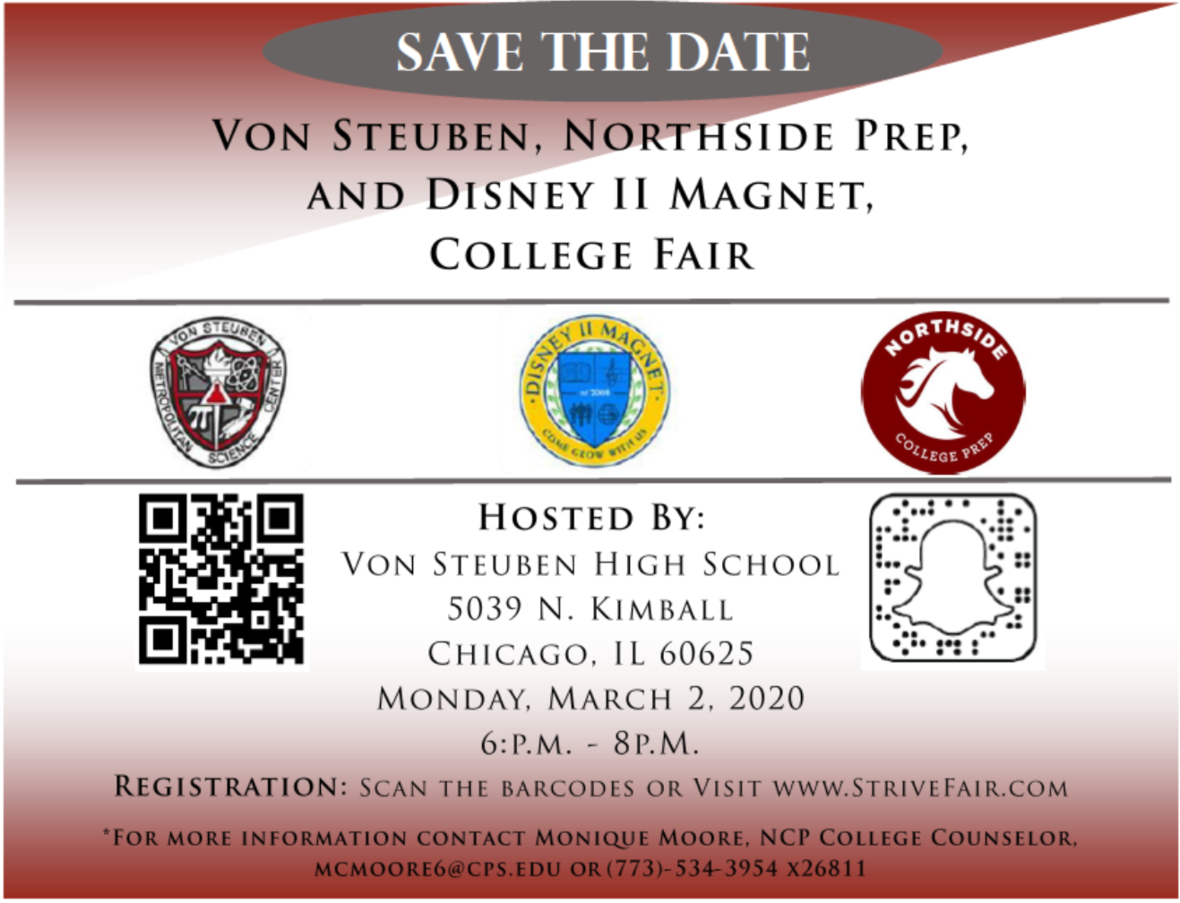# Joint College Fair Planned

Monday, March 2
6:00-8:00 pm
Von Steuben High School, 5039 N. Kimball

Northside Prep, Disney II Magnet, and Von Steuben High School will be hosting a Joint College Fair. Students should visit www.strivefair.com or scan the barcode above to avoid the long lines the day of the college fair! For step-by-step instructions to register for the college fair, CLICK HERE.

Click HERE to see the list of post-secondary pathways that will be presented at the college fair:

• Four Year Colleges
• Two Year Colleges
• International Colleges
• Gap Year Programs
• Military Branches

To prepare for the upcoming college fair, CLICK HERE to review the checklist for best practices before, during, and after the college fair. CLICK HERE to identify or brainstorm potential questions you may have for the representatives.

For more information about the fair, contact Ms. Moore, Northside Prep College Counselor, at mcmoore6@cps.edu or (773)-534-3954 x26811.

# CPS Scholarship Notifications and College Events Around the City

For more details about the upcoming events and to access the most recent updates, click HERE to view the College Calendar on the Northside Prep Counseling Website. Also, for additional scholarship opportunities visit the Northside College Prep Counseling page, click HERE.

Saturday, February 1

▪ App Opens: Chicago Botanic Garden: College First Internship Program
▪ App Opens: Discover Student Loans Scholarship Award
▪ App Opens: Japanese American Service Committee (JASC) Scholarship
▪ App Opens: Omega Psi Phi Fraternity, Inc. - Iota Chapter Scholarship
 ▪ App Opens: Chicago Botanic Garden: College First Internship Program
 ▪ App Opens: Discover Student Loans Scholarship Award
 ▪ App Opens: Japanese American Service Committee (JASC) Scholarship
 ▪ App Opens: Omega Psi Phi Fraternity, Inc. - Iota Chapter Scholarship

Sunday, February 2

▪ App Opens: AKArama Foundation Scholarships - Alpha Kappa Alpha Sorority Inc - Theta Omega Chapter
 ▪ App Opens: AKArama Foundation Scholarships - Alpha Kappa Alpha Sorority Inc - Theta Omega Chapter

Monday, February 3

▪ App Opens: Calculated Genius Steminist Scholarship Program
▪ App Opens: CBC Spouses Education Scholarship
▪ App Opens: CBC Spouses Performing Arts Scholarship
▪ App Opens: CBC Spouses Visual Arts Scholarship
 ▪ App Opens: Calculated Genius Steminist Scholarship Program
 ▪ App Opens: CBC Spouses Education Scholarship
 ▪ App Opens: CBC Spouses Performing Arts Scholarship
 ▪ App Opens: CBC Spouses Visual Arts Scholarship

Wednesday, February 5

▪ App Closes: Global Citizen Year Fellowship
▪ App Closes: McDonald’s HACER® Program
 ▪ App Closes: Global Citizen Year Fellowship
 ▪ App Closes: McDonald’s HACER® Program

Friday, February 7

▪ App Closes: AMS Freshman Undergraduate Scholarship
▪ App Closes: AMS Minority Scholarship
▪ App Closes: Illinois School Counselor Association (ISCA) - Student Scholarship
▪ App Closes: Planning Your Success Scholarship
▪ App Closes: Wentcher Foundation Scholarship
▪ App Opens: The Marcus J. Bradshaw Foundation Scholarship
 ▪ App Closes: AMS Freshman Undergraduate Scholarship
 ▪ App Closes: AMS Minority Scholarship
 ▪ App Closes: Illinois School Counselor Association (ISCA) - Student Scholarship
 ▪ App Closes: Planning Your Success Scholarship
 ▪ App Closes: Wentcher Foundation Scholarship
 ▪ App Opens: The Marcus J. Bradshaw Foundation Scholarship

Wednesday, February 12

▪ App Closes: Iota Phi Lambda Sorority, Inc. - Dorethea N. Hornbuckle Scholarship
 ▪ App Closes: Iota Phi Lambda Sorority, Inc. - Dorethea N. Hornbuckle Scholarship

Friday, February 14

▪ App Closes: Illinois PTA Lillian E. Glover Scholarship Program
 ▪ App Closes: Illinois PTA Lillian E. Glover Scholarship Program

Saturday, February 15

▪ App Closes: Hispanic Scholarship Fund (HSF) General Scholarship
▪ App Closes: National Co-Op Scholarship
▪ App Closes: Xi Lambda Educational Foundation Scholarship
 ▪ App Closes: Hispanic Scholarship Fund (HSF) General Scholarship
 ▪ App Closes: National Co-Op Scholarship
 ▪ App Closes: Xi Lambda Educational Foundation Scholarship

Monday, February 17

▪ App Closes: Princeton University Summer Journalism Program
 ▪ App Closes: Princeton University Summer Journalism Program

Friday, February 21

▪ App Closes: AKArama Foundation Scholarships - Alpha Kappa Alpha Sorority Inc - Theta Omega Chapter
▪ App Closes: McKinsey Diverse Young Leaders Scholarship
▪ App Closes: Midwest Great Lakes Regional Scholarship
▪ App Closes: National Association of Hispanic Journalists Educational Programs
 ▪ App Closes: AKArama Foundation Scholarships - Alpha Kappa Alpha Sorority Inc - Theta Omega Chapter
 ▪ App Closes: McKinsey Diverse Young Leaders Scholarship
 ▪ App Closes: Midwest Great Lakes Regional Scholarship
 ▪ App Closes: National Association of Hispanic Journalists Educational Programs

Thursday, February 27

▪ App Closes: The Dream.US Scholarship
 ▪ App Closes: The Dream.US Scholarship

Friday, February 28

▪ App Closes: Elks National Oratorical Contest
 ▪ App Closes: Elks National Oratorical Contest

Saturday, February 29

▪ App Closes: College Board Opportunity Scholarships
 ▪ App Closes: College Board Opportunity Scholarships
 table div table+table+table+table+table+table+table+table+table+table+table+table+table+table+table+table+table+table+table+table+table+table+table+table+table+table+table+table+table+table+table+table+table+table+table+table+table+table+table+table+table+table+table+table+table+table+table+table+table+table+table+table+table+table+table+table+table+table+table+table+table div table{width:100%;padding:0}table div table+table+table+table+table+table+table+table+table+table+table+table+table+table+table+table+table+table+table+table+table+table+table+table+table+table+table+table+table+table+table+table+table+table+table+table+table+table+table+table+table+table+table+table+table+table+table+table+table+table+table+table+table+table+table+table+table+table+table+table+table div table img{width:96.23%;padding:0;float:none}table div table+table+table+table+table+table+table+table+table+table+table+table+table+table+table+table+table+table+table+table+table+table+table+table+table+table+table+table+table+table+table+table+table+table+table+table+table+table+table+table+table+table+table+table+table+table+table+table+table+table+table+table+table+table+table+table+table+table+table+table+table div table td{width:100%;padding:0 1.88% 18px}/* styles */## Important Dates for the Class of 2020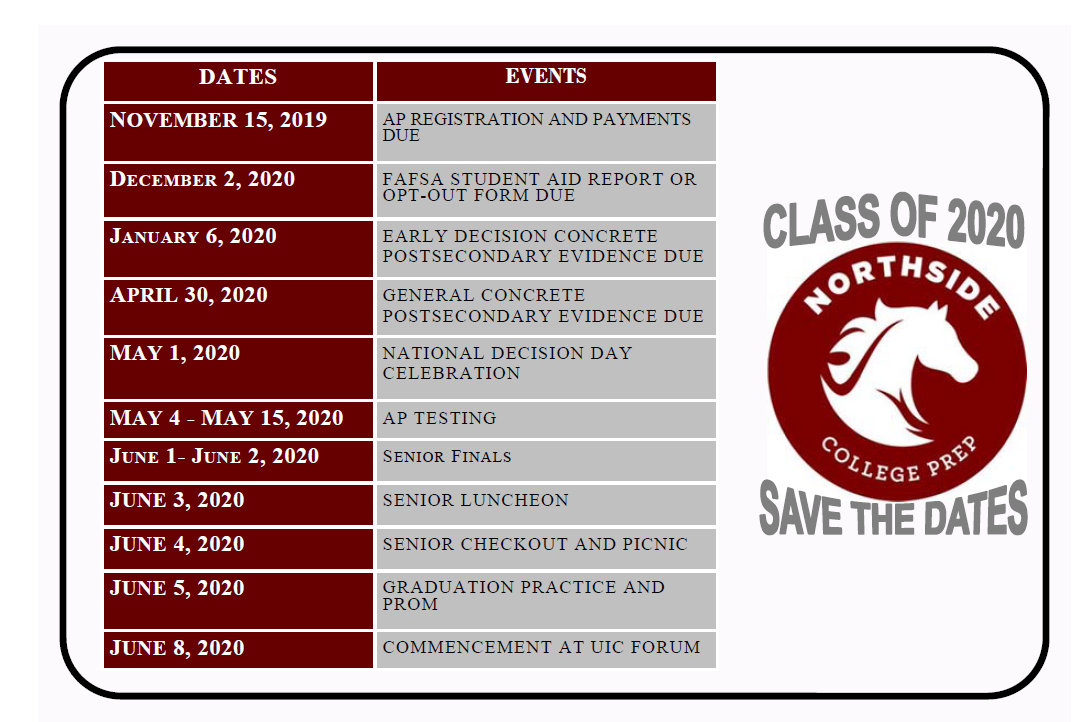table div table+table+table+table+table+table+table+table+table+table+table+table+table+table+table+table+table+table+table+table+table+table+table+table+table+table+table+table+table+table+table+table+table+table+table+table+table+table+table+table+table+table+table+table+table+table+table+table+table+table+table+table+table+table+table+table+table+table+table+table+table+table+table+table div table{width:100%;padding:0}table div table+table+table+table+table+table+table+table+table+table+table+table+table+table+table+table+table+table+table+table+table+table+table+table+table+table+table+table+table+table+table+table+table+table+table+table+table+table+table+table+table+table+table+table+table+table+table+table+table+table+table+table+table+table+table+table+table+table+table+table+table+table+table+table div table img{width:96.23%;padding:0;float:none}table div table+table+table+table+table+table+table+table+table+table+table+table+table+table+table+table+table+table+table+table+table+table+table+table+table+table+table+table+table+table+table+table+table+table+table+table+table+table+table+table+table+table+table+table+table+table+table+table+table+table+table+table+table+table+table+table+table+table+table+table+table+table+table+table div table td{width:100%;padding:0 1.88% 18px}/* styles */## Important Dates for the Class of 2021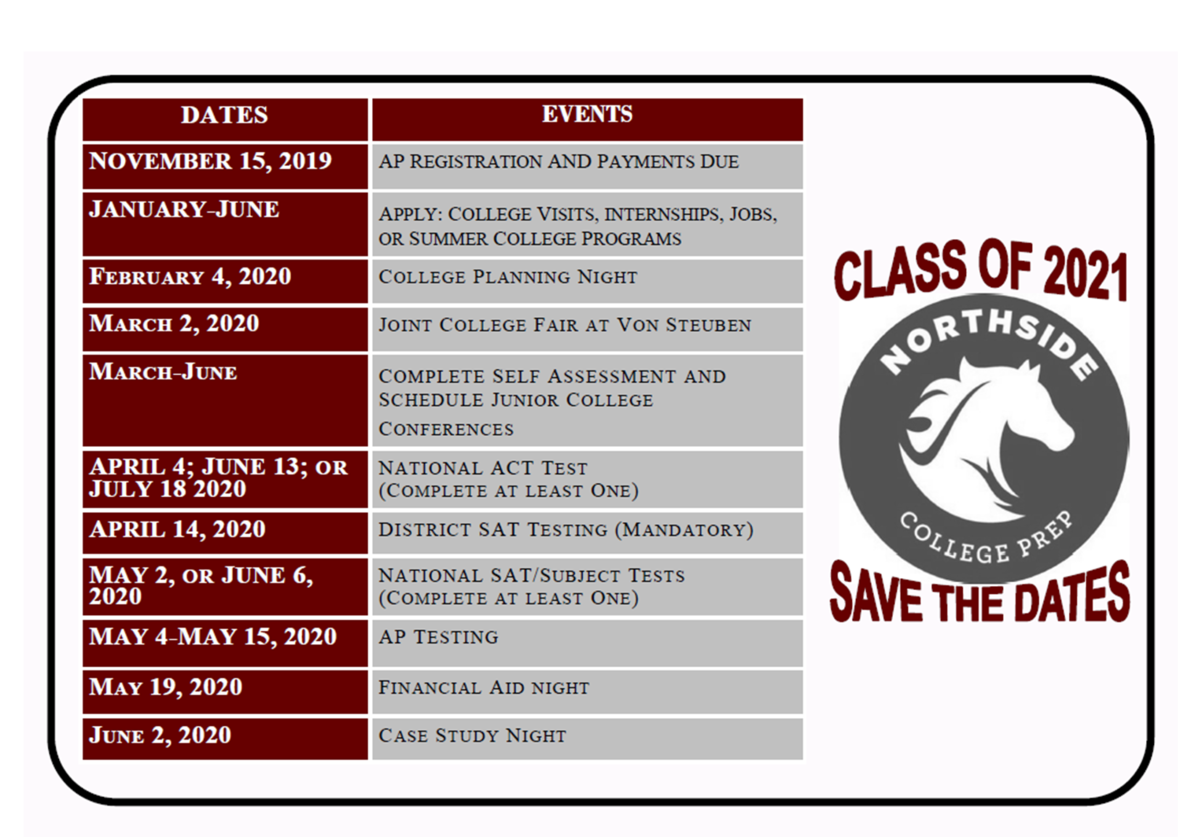table div table+table+table+table+table+table+table+table+table+table+table+table+table+table+table+table+table+table+table+table+table+table+table+table+table+table+table+table+table+table+table+table+table+table+table+table+table+table+table+table+table+table+table+table+table+table+table+table+table+table+table+table+table+table+table+table+table+table+table+table+table+table+table+table+table+table+table div table{width:100%;padding:0}table div table+table+table+table+table+table+table+table+table+table+table+table+table+table+table+table+table+table+table+table+table+table+table+table+table+table+table+table+table+table+table+table+table+table+table+table+table+table+table+table+table+table+table+table+table+table+table+table+table+table+table+table+table+table+table+table+table+table+table+table+table+table+table+table+table+table+table div table img{width:96.23%;padding:0;float:none}table div table+table+table+table+table+table+table+table+table+table+table+table+table+table+table+table+table+table+table+table+table+table+table+table+table+table+table+table+table+table+table+table+table+table+table+table+table+table+table+table+table+table+table+table+table+table+table+table+table+table+table+table+table+table+table+table+table+table+table+table+table+table+table+table+table+table+table div table td{width:100%;padding:0 1.88% 18px}/* styles */# New to Parent Portal?

1. Obtain your student's PIN, which should be on your acceptance letter or school welcome letter.
2. Create an account HERE.
 1 Obtain your student's PIN, which should be on your acceptance letter or school welcome letter.
 2 Create an account HERE.

PINs can only be provided to the parent/guardian in person or by mail to the address on file. They cannot be provided by phone or email, or to a student. To request your PIN, please contact Sonia Fernandez in the Counseling Office.

 table div table+table+table+table+table+table+table+table+table+table+table+table+table+table+table+table+table+table+table+table+table+table+table+table+table+table+table+table+table+table+table+table+table+table+table+table+table+table+table+table+table+table+table+table+table+table+table+table+table+table+table+table+table+table+table+table+table+table+table+table+table+table+table+table+table+table+table+table+table div table{width:100%;padding:0}table div table+table+table+table+table+table+table+table+table+table+table+table+table+table+table+table+table+table+table+table+table+table+table+table+table+table+table+table+table+table+table+table+table+table+table+table+table+table+table+table+table+table+table+table+table+table+table+table+table+table+table+table+table+table+table+table+table+table+table+table+table+table+table+table+table+table+table+table+table div table img{width:96.23%;padding:0;float:none}table div table+table+table+table+table+table+table+table+table+table+table+table+table+table+table+table+table+table+table+table+table+table+table+table+table+table+table+table+table+table+table+table+table+table+table+table+table+table+table+table+table+table+table+table+table+table+table+table+table+table+table+table+table+table+table+table+table+table+table+table+table+table+table+table+table+table+table+table+table div table td{width:100%;padding:0 1.88% 18px}/* styles */# CPS Volunteer Requirements

According to CPS policy, anybody who is going to be in contact with students must be approved as a CPS volunteer. There are two levels of volunteers, Level 1 and Level 2.

## Level I Volunteers

(Please allow 8-12 weeks for the entire process)
A fingerprint criminal background check is required for all Level I Volunteers.

A Level I volunteer is:

1. A parent providing volunteer service in their child’s school or classroom for 10 or more hours/week;
2. An individual providing volunteer service for 5 or more hours/week at a school where s/he does not have a child enrolled;
3. Any individual chaperoning an overnight school-sponsored trip, regardless of the hours/week that the volunteer serves (See also the Student Travel Policy).
 1 A parent providing volunteer service in their child’s school or classroom for 10 or more hours/week;
 2 An individual providing volunteer service for 5 or more hours/week at a school where s/he does not have a child enrolled;
 3 Any individual chaperoning an overnight school-sponsored trip, regardless of the hours/week that the volunteer serves (See also the Student Travel Policy).

## Level II Volunteers

(This process can be completed in a day & is required even of volunteers for Friends of Northside events)
A fingerprint criminal background check is not required for Level II Volunteers.

A Level II Volunteer is:

1. A parent providing supervised volunteer service in their child’s school or classroom for less than 10 hours/week;
2. Any individual providing volunteer service for less than 5 hours/week at a school where s/he does not have a child enrolled;
3. An individual providing incidental volunteer service with no ongoing individualized interaction with a student(s) including those who speak at a class/assembly, judge academic competitions, give musical performance, participate in the “Principal for a Day” program, job shadowing event or other one-time event provided where there is direct supervision of the activity/event by regular school employees;
4. A parent accompanying his/her child’s class on a one-day field trip or another type of extracurricular activity that does not involve an overnight stay;
5. An individual providing volunteer service on projects/activities involving no or nominal contact with children (home-based volunteer activities, volunteers serving at Central or Network office).
 1 A parent providing supervised volunteer service in their child’s school or classroom for less than 10 hours/week;
 2 Any individual providing volunteer service for less than 5 hours/week at a school where s/he does not have a child enrolled;
 3 An individual providing incidental volunteer service with no ongoing individualized interaction with a student(s) including those who speak at a class/assembly, judge academic competitions, give musical performance, participate in the “Principal for a Day” program, job shadowing event or other one-time event provided where there is direct supervision of the activity/event by regular school employees;
 4 A parent accompanying his/her child’s class on a one-day field trip or another type of extracurricular activity that does not involve an overnight stay;
 5 An individual providing volunteer service on projects/activities involving no or nominal contact with children (home-based volunteer activities, volunteers serving at Central or Network office).

To become a volunteer, please visit CPS' Volunteer website and follow the instructions. There is also a link on Northside's website on the Parents page Northside Volunteers.

Assistant Principal, Kaitlin O'Hare is Northside's Volunteer Coordinator. After applying to become a volunteer, you must visit the school and show Ms. O'Hare your ID, so she can approve you to move forward in the volunteer process. For Level II volunteers, that is the only step you must take. For Level 1, you will receive further instructions from CPS.

 table div table+table+table+table+table+table+table+table+table+table+table+table+table+table+table+table+table+table+table+table+table+table+table+table+table+table+table+table+table+table+table+table+table+table+table+table+table+table+table+table+table+table+table+table+table+table+table+table+table+table+table+table+table+table+table+table+table+table+table+table+table+table+table+table+table+table+table+table+table+table+table div table{width:100%;padding:0}table div table+table+table+table+table+table+table+table+table+table+table+table+table+table+table+table+table+table+table+table+table+table+table+table+table+table+table+table+table+table+table+table+table+table+table+table+table+table+table+table+table+table+table+table+table+table+table+table+table+table+table+table+table+table+table+table+table+table+table+table+table+table+table+table+table+table+table+table+table+table+table div table img{width:96.23%;padding:0;float:none}table div table+table+table+table+table+table+table+table+table+table+table+table+table+table+table+table+table+table+table+table+table+table+table+table+table+table+table+table+table+table+table+table+table+table+table+table+table+table+table+table+table+table+table+table+table+table+table+table+table+table+table+table+table+table+table+table+table+table+table+table+table+table+table+table+table+table+table+table+table+table+table div table td{width:100%;padding:0 1.88% 18px}/* styles */# FON Connect

Friends of Northside uses a customer/constituent relationship management (CRM) system known as FON Connect that allows our parent volunteer organization to work from one database for events. It is not a CPS database and is completely different and separate from the CPS Parent Portal and Northside's EPay website.

FON Connect also serves as the NCP Family Directory and allows users to review their donation history, update their contact information, register for upcoming events, and more. Each parent has a separate account. Transactions, including donations, are recorded in the account of the parent who initiated the transaction or donation.

If you’re new to FON Connect, you'll need to set up a login and password. To do so:

 table div table+table+table+table+table+table+table+table+table+table+table+table+table+table+table+table+table+table+table+table+table+table+table+table+table+table+table+table+table+table+table+table+table+table+table+table+table+table+table+table+table+table+table+table+table+table+table+table+table+table+table+table+table+table+table+table+table+table+table+table+table+table+table+table+table+table+table+table+table+table+table+table+table div table{width:100%;padding:0}table div table+table+table+table+table+table+table+table+table+table+table+table+table+table+table+table+table+table+table+table+table+table+table+table+table+table+table+table+table+table+table+table+table+table+table+table+table+table+table+table+table+table+table+table+table+table+table+table+table+table+table+table+table+table+table+table+table+table+table+table+table+table+table+table+table+table+table+table+table+table+table+table+table div table img{width:96.23%;padding:0;float:none}table div table+table+table+table+table+table+table+table+table+table+table+table+table+table+table+table+table+table+table+table+table+table+table+table+table+table+table+table+table+table+table+table+table+table+table+table+table+table+table+table+table+table+table+table+table+table+table+table+table+table+table+table+table+table+table+table+table+table+table+table+table+table+table+table+table+table+table+table+table+table+table+table+table div table td{width:100%;padding:0 1.88% 18px}/* styles */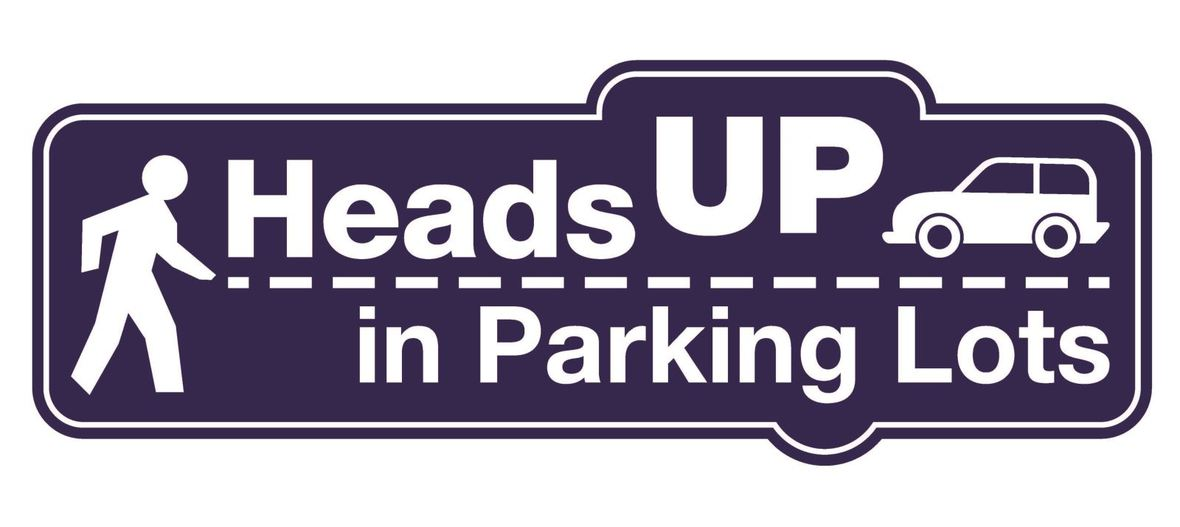# Parking Lot Safety

Our NCP school parking lot is a bustling place before and after school. Families dropping off and picking up students are asked to be mindful of both safety and courtesy. Please assist us by ensuring that traffic lanes stay clear during drop-off and pick-up times and by following the parking lot procedures.

# Parking Lot Procedures

ALL vehicles MUST exit onto Kedzie. You may enter the parking lot from Kedzie or Bryn Mawr, but regardless of where you enter, all cars must exit onto Kedzie. For the safety of our students and the rest of the Northside community, please do not exit onto Bryn Mawr.

The first few days will be busy at drop-off and pick-up. Please, for the safety of all involved, plan to give yourself enough time for drop-off and pick-up and please be patient while in the parking lot. Pulling over to the curb on the right as your student enters or exits your vehicle keeps students safe and traffic flow efficient. Our safety team will be actively working to ensure that students arrive and depart safely each day and that vehicles in school lots remain damage-free.

 table div table+table+table+table+table+table+table+table+table+table+table+table+table+table+table+table+table+table+table+table+table+table+table+table+table+table+table+table+table+table+table+table+table+table+table+table+table+table+table+table+table+table+table+table+table+table+table+table+table+table+table+table+table+table+table+table+table+table+table+table+table+table+table+table+table+table+table+table+table+table+table+table+table+table+table+table div table{width:100%;padding:0}table div table+table+table+table+table+table+table+table+table+table+table+table+table+table+table+table+table+table+table+table+table+table+table+table+table+table+table+table+table+table+table+table+table+table+table+table+table+table+table+table+table+table+table+table+table+table+table+table+table+table+table+table+table+table+table+table+table+table+table+table+table+table+table+table+table+table+table+table+table+table+table+table+table+table+table+table div table img{width:96.23%;padding:0;float:none}table div table+table+table+table+table+table+table+table+table+table+table+table+table+table+table+table+table+table+table+table+table+table+table+table+table+table+table+table+table+table+table+table+table+table+table+table+table+table+table+table+table+table+table+table+table+table+table+table+table+table+table+table+table+table+table+table+table+table+table+table+table+table+table+table+table+table+table+table+table+table+table+table+table+table+table+table div table td{width:100%;padding:0 1.88% 18px}/* styles */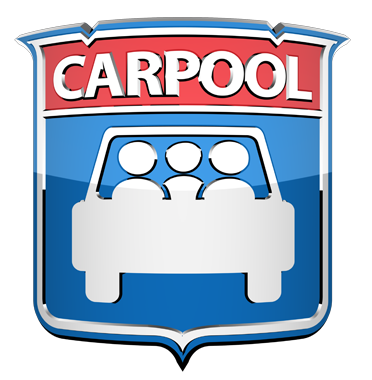# NCP Carpool Connection!

If you're interested in carpooling to school, add your address to the 2019-2020 NCP Carpool map and reach out to contact a potential carpool family on the map. Find the map HERE or on the Welcome page of FON Connect.

 table div table+table+table+table+table+table+table+table+table+table+table+table+table+table+table+table+table+table+table+table+table+table+table+table+table+table+table+table+table+table+table+table+table+table+table+table+table+table+table+table+table+table+table+table+table+table+table+table+table+table+table+table+table+table+table+table+table+table+table+table+table+table+table+table+table+table+table+table+table+table+table+table+table+table+table+table+table+table div table{width:100%;padding:0}table div table+table+table+table+table+table+table+table+table+table+table+table+table+table+table+table+table+table+table+table+table+table+table+table+table+table+table+table+table+table+table+table+table+table+table+table+table+table+table+table+table+table+table+table+table+table+table+table+table+table+table+table+table+table+table+table+table+table+table+table+table+table+table+table+table+table+table+table+table+table+table+table+table+table+table+table+table+table div table img{width:96.23%;padding:0;float:none}table div table+table+table+table+table+table+table+table+table+table+table+table+table+table+table+table+table+table+table+table+table+table+table+table+table+table+table+table+table+table+table+table+table+table+table+table+table+table+table+table+table+table+table+table+table+table+table+table+table+table+table+table+table+table+table+table+table+table+table+table+table+table+table+table+table+table+table+table+table+table+table+table+table+table+table+table+table+table div table td{width:100%;padding:0 1.88% 18px}/* styles */# Attention, Parents of CTA Commuters

Did you know that the #82 and #92 buses pick up students in front of Northside at the end of the school day? The Kimball Brown line station is also close to school at Kimball and Lawrence.

These are just a few of the ways students can use public transportation to commute to school. More information on routes for your student can be found at the CTA website HERE.

 table div table+table+table+table+table+table+table+table+table+table+table+table+table+table+table+table+table+table+table+table+table+table+table+table+table+table+table+table+table+table+table+table+table+table+table+table+table+table+table+table+table+table+table+table+table+table+table+table+table+table+table+table+table+table+table+table+table+table+table+table+table+table+table+table+table+table+table+table+table+table+table+table+table+table+table+table+table+table+table+table div table{width:100%;padding:0}table div table+table+table+table+table+table+table+table+table+table+table+table+table+table+table+table+table+table+table+table+table+table+table+table+table+table+table+table+table+table+table+table+table+table+table+table+table+table+table+table+table+table+table+table+table+table+table+table+table+table+table+table+table+table+table+table+table+table+table+table+table+table+table+table+table+table+table+table+table+table+table+table+table+table+table+table+table+table+table+table div table img{width:96.23%;padding:0;float:none}table div table+table+table+table+table+table+table+table+table+table+table+table+table+table+table+table+table+table+table+table+table+table+table+table+table+table+table+table+table+table+table+table+table+table+table+table+table+table+table+table+table+table+table+table+table+table+table+table+table+table+table+table+table+table+table+table+table+table+table+table+table+table+table+table+table+table+table+table+table+table+table+table+table+table+table+table+table+table+table+table div table td{width:100%;padding:0 1.88% 18px}/* styles */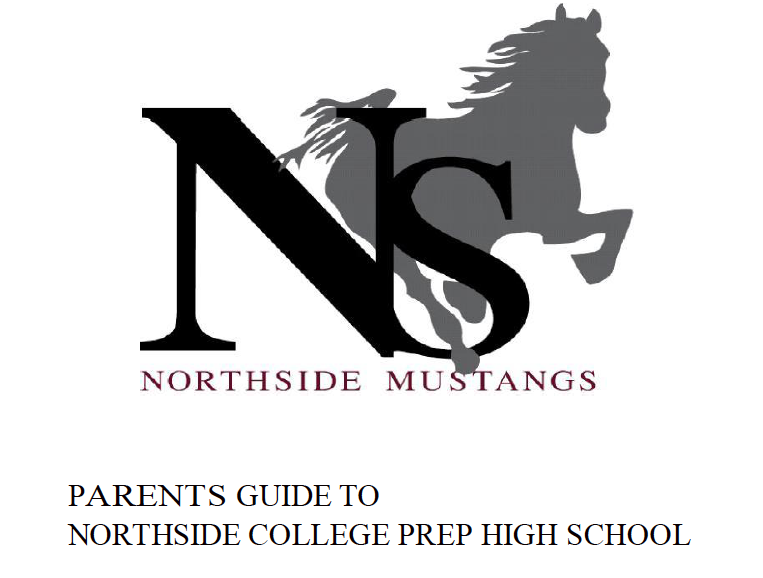# Navigating Northside

Navigating Northside, our parent handbook, is available on the school website under the "Parents" tab. Click HERE to access it directly.

 table div table+table+table+table+table+table+table+table+table+table+table+table+table+table+table+table+table+table+table+table+table+table+table+table+table+table+table+table+table+table+table+table+table+table+table+table+table+table+table+table+table+table+table+table+table+table+table+table+table+table+table+table+table+table+table+table+table+table+table+table+table+table+table+table+table+table+table+table+table+table+table+table+table+table+table+table+table+table+table+table+table+table div table{width:100%;padding:0}table div table+table+table+table+table+table+table+table+table+table+table+table+table+table+table+table+table+table+table+table+table+table+table+table+table+table+table+table+table+table+table+table+table+table+table+table+table+table+table+table+table+table+table+table+table+table+table+table+table+table+table+table+table+table+table+table+table+table+table+table+table+table+table+table+table+table+table+table+table+table+table+table+table+table+table+table+table+table+table+table+table+table div table img{width:96.23%;padding:0;float:none}table div table+table+table+table+table+table+table+table+table+table+table+table+table+table+table+table+table+table+table+table+table+table+table+table+table+table+table+table+table+table+table+table+table+table+table+table+table+table+table+table+table+table+table+table+table+table+table+table+table+table+table+table+table+table+table+table+table+table+table+table+table+table+table+table+table+table+table+table+table+table+table+table+table+table+table+table+table+table+table+table+table+table div table td{width:100%;padding:0 1.88% 18px}/* styles *//* styles */ Check out The HoofBeat, Northside's online student newspaper, to get NCP news from the student perspective!
 table div table+table+table+table+table+table+table+table+table+table+table+table+table+table+table+table+table+table+table+table+table+table+table+table+table+table+table+table+table+table+table+table+table+table+table+table+table+table+table+table+table+table+table+table+table+table+table+table+table+table+table+table+table+table+table+table+table+table+table+table+table+table+table+table+table+table+table+table+table+table+table+table+table+table+table+table+table+table+table+table+table+table+table+table+table div table{width:100%;padding:0}table div table+table+table+table+table+table+table+table+table+table+table+table+table+table+table+table+table+table+table+table+table+table+table+table+table+table+table+table+table+table+table+table+table+table+table+table+table+table+table+table+table+table+table+table+table+table+table+table+table+table+table+table+table+table+table+table+table+table+table+table+table+table+table+table+table+table+table+table+table+table+table+table+table+table+table+table+table+table+table+table+table+table+table+table+table div table img{width:96.23%;padding:0;float:none}table div table+table+table+table+table+table+table+table+table+table+table+table+table+table+table+table+table+table+table+table+table+table+table+table+table+table+table+table+table+table+table+table+table+table+table+table+table+table+table+table+table+table+table+table+table+table+table+table+table+table+table+table+table+table+table+table+table+table+table+table+table+table+table+table+table+table+table+table+table+table+table+table+table+table+table+table+table+table+table+table+table+table+table+table+table div table td{width:100%;padding:0 1.88% 18px}/* styles */# Check out the FAN!

FAN is Family Action Network, an organization which connects parents, educators and professionals through collaborative programming that educates, inspires and positively impacts the broader community. FAN hosts speaker events, discussing the issues that interest you.

FAN events are free to all.

 table div table+table+table+table+table+table+table+table+table+table+table+table+table+table+table+table+table+table+table+table+table+table+table+table+table+table+table+table+table+table+table+table+table+table+table+table+table+table+table+table+table+table+table+table+table+table+table+table+table+table+table+table+table+table+table+table+table+table+table+table+table+table+table+table+table+table+table+table+table+table+table+table+table+table+table+table+table+table+table+table+table+table+table+table+table+table+table div table{width:100%;padding:0}table div table+table+table+table+table+table+table+table+table+table+table+table+table+table+table+table+table+table+table+table+table+table+table+table+table+table+table+table+table+table+table+table+table+table+table+table+table+table+table+table+table+table+table+table+table+table+table+table+table+table+table+table+table+table+table+table+table+table+table+table+table+table+table+table+table+table+table+table+table+table+table+table+table+table+table+table+table+table+table+table+table+table+table+table+table+table+table div table img{width:96.23%;padding:0;float:none}table div table+table+table+table+table+table+table+table+table+table+table+table+table+table+table+table+table+table+table+table+table+table+table+table+table+table+table+table+table+table+table+table+table+table+table+table+table+table+table+table+table+table+table+table+table+table+table+table+table+table+table+table+table+table+table+table+table+table+table+table+table+table+table+table+table+table+table+table+table+table+table+table+table+table+table+table+table+table+table+table+table+table+table+table+table+table+table div table td{width:100%;padding:0 1.88% 18px}/* styles */# Help Northside When You Shop Online

## You can help our school with no extra cost to you.

AmazonSmile is a simple and automatic way to support Northside while you shop.

To choose Northside, please note that our AmazonSmile name is Friends of "North" "Side" (North Side - two words at AmazonSmile).

 table div table+table+table+table+table+table+table+table+table+table+table+table+table+table+table+table+table+table+table+table+table+table+table+table+table+table+table+table+table+table+table+table+table+table+table+table+table+table+table+table+table+table+table+table+table+table+table+table+table+table+table+table+table+table+table+table+table+table+table+table+table+table+table+table+table+table+table+table+table+table+table+table+table+table+table+table+table+table+table+table+table+table+table+table+table+table+table+table+table+table div table{width:100%;padding:0}table div table+table+table+table+table+table+table+table+table+table+table+table+table+table+table+table+table+table+table+table+table+table+table+table+table+table+table+table+table+table+table+table+table+table+table+table+table+table+table+table+table+table+table+table+table+table+table+table+table+table+table+table+table+table+table+table+table+table+table+table+table+table+table+table+table+table+table+table+table+table+table+table+table+table+table+table+table+table+table+table+table+table+table+table+table+table+table+table+table+table div table img{width:96.23%;padding:0;float:none}table div table+table+table+table+table+table+table+table+table+table+table+table+table+table+table+table+table+table+table+table+table+table+table+table+table+table+table+table+table+table+table+table+table+table+table+table+table+table+table+table+table+table+table+table+table+table+table+table+table+table+table+table+table+table+table+table+table+table+table+table+table+table+table+table+table+table+table+table+table+table+table+table+table+table+table+table+table+table+table+table+table+table+table+table+table+table+table+table+table+table div table td{width:100%;padding:0 1.88% 18px}/* styles */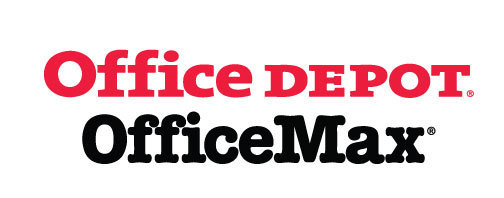# Give Back to Northside

Purchase school and office supplies at Office Depot and OfficeMax and Northside can receive 5% back in credits or FREE supplies. Simply provide our school ID 70031171 at checkout, online, or in stores. There’s no cost to you!

 table div table+table+table+table+table+table+table+table+table+table+table+table+table+table+table+table+table+table+table+table+table+table+table+table+table+table+table+table+table+table+table+table+table+table+table+table+table+table+table+table+table+table+table+table+table+table+table+table+table+table+table+table+table+table+table+table+table+table+table+table+table+table+table+table+table+table+table+table+table+table+table+table+table+table+table+table+table+table+table+table+table+table+table+table+table+table+table+table+table+table+table+table div table{width:100%;padding:0}table div table+table+table+table+table+table+table+table+table+table+table+table+table+table+table+table+table+table+table+table+table+table+table+table+table+table+table+table+table+table+table+table+table+table+table+table+table+table+table+table+table+table+table+table+table+table+table+table+table+table+table+table+table+table+table+table+table+table+table+table+table+table+table+table+table+table+table+table+table+table+table+table+table+table+table+table+table+table+table+table+table+table+table+table+table+table+table+table+table+table+table+table div table img{width:96.23%;padding:0;float:none}table div table+table+table+table+table+table+table+table+table+table+table+table+table+table+table+table+table+table+table+table+table+table+table+table+table+table+table+table+table+table+table+table+table+table+table+table+table+table+table+table+table+table+table+table+table+table+table+table+table+table+table+table+table+table+table+table+table+table+table+table+table+table+table+table+table+table+table+table+table+table+table+table+table+table+table+table+table+table+table+table+table+table+table+table+table+table+table+table+table+table+table+table div table td{width:100%;padding:0 1.88% 18px}/* styles */# Follow Us on Social Media

▪ Northside Prep Parents Facebook Page, a closed group for parents, by parents, to share their knowledge of Northside
▪ Friends of Northside Facebook Page, for news everyone can view
 ▪ Northside Prep Parents Facebook Page, a closed group for parents, by parents, to share their knowledge of Northside
 ▪ Friends of Northside Facebook Page, for news everyone can view
 table div table+table+table+table+table+table+table+table+table+table+table+table+table+table+table+table+table+table+table+table+table+table+table+table+table+table+table+table+table+table+table+table+table+table+table+table+table+table+table+table+table+table+table+table+table+table+table+table+table+table+table+table+table+table+table+table+table+table+table+table+table+table+table+table+table+table+table+table+table+table+table+table+table+table+table+table+table+table+table+table+table+table+table+table+table+table+table+table+table+table+table+table+table+table div table{width:100%;padding:0}table div table+table+table+table+table+table+table+table+table+table+table+table+table+table+table+table+table+table+table+table+table+table+table+table+table+table+table+table+table+table+table+table+table+table+table+table+table+table+table+table+table+table+table+table+table+table+table+table+table+table+table+table+table+table+table+table+table+table+table+table+table+table+table+table+table+table+table+table+table+table+table+table+table+table+table+table+table+table+table+table+table+table+table+table+table+table+table+table+table+table+table+table+table+table div table img{width:96.23%;padding:0;float:none}table div table+table+table+table+table+table+table+table+table+table+table+table+table+table+table+table+table+table+table+table+table+table+table+table+table+table+table+table+table+table+table+table+table+table+table+table+table+table+table+table+table+table+table+table+table+table+table+table+table+table+table+table+table+table+table+table+table+table+table+table+table+table+table+table+table+table+table+table+table+table+table+table+table+table+table+table+table+table+table+table+table+table+table+table+table+table+table+table+table+table+table+table+table+table div table td{width:100%;padding:0 1.88% 18px}/* styles */Northside’s website can be found HERE.

Friends of Northside's website can be found HERE.

 table div table+table+table+table+table+table+table+table+table+table+table+table+table+table+table+table+table+table+table+table+table+table+table+table+table+table+table+table+table+table+table+table+table+table+table+table+table+table+table+table+table+table+table+table+table+table+table+table+table+table+table+table+table+table+table+table+table+table+table+table+table+table+table+table+table+table+table+table+table+table+table+table+table+table+table+table+table+table+table+table+table+table+table+table+table+table+table+table+table+table+table+table+table+table+table+table div table{width:100%;padding:0}table div table+table+table+table+table+table+table+table+table+table+table+table+table+table+table+table+table+table+table+table+table+table+table+table+table+table+table+table+table+table+table+table+table+table+table+table+table+table+table+table+table+table+table+table+table+table+table+table+table+table+table+table+table+table+table+table+table+table+table+table+table+table+table+table+table+table+table+table+table+table+table+table+table+table+table+table+table+table+table+table+table+table+table+table+table+table+table+table+table+table+table+table+table+table+table+table div table img{width:96.23%;padding:0;float:none}table div table+table+table+table+table+table+table+table+table+table+table+table+table+table+table+table+table+table+table+table+table+table+table+table+table+table+table+table+table+table+table+table+table+table+table+table+table+table+table+table+table+table+table+table+table+table+table+table+table+table+table+table+table+table+table+table+table+table+table+table+table+table+table+table+table+table+table+table+table+table+table+table+table+table+table+table+table+table+table+table+table+table+table+table+table+table+table+table+table+table+table+table+table+table+table+table div table td{width:100%;padding:0 1.88% 18px}/* styles */